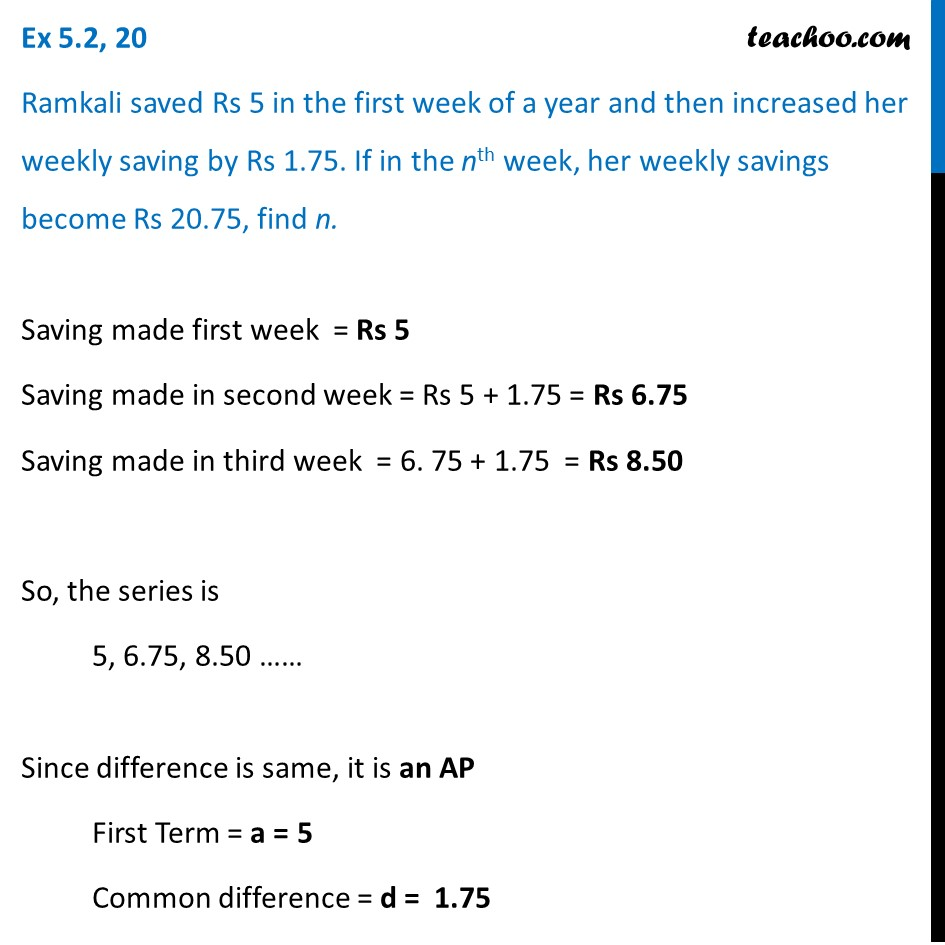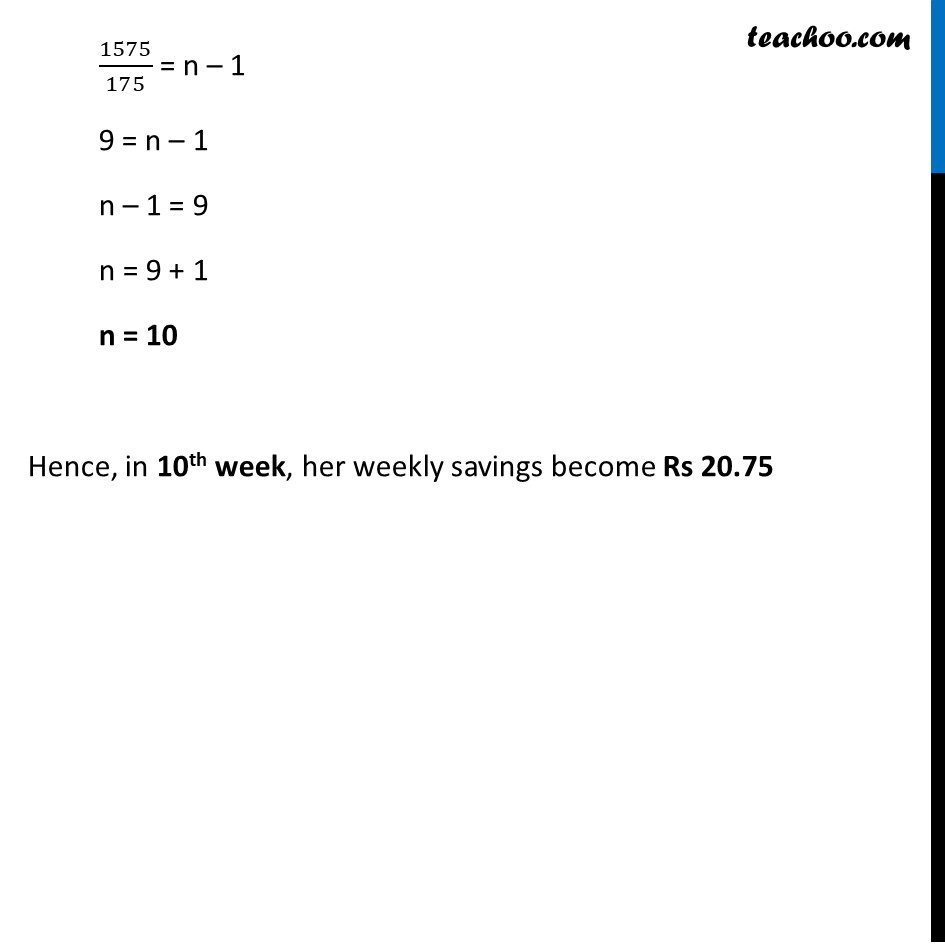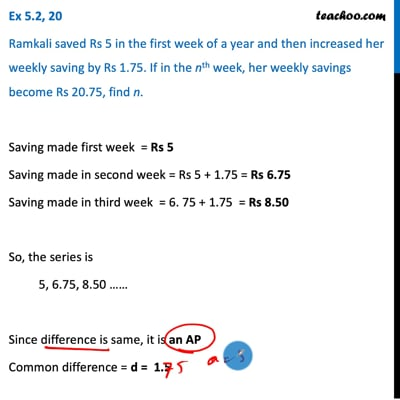Ex 5.2

Chapter 5 Class 10 Arithmetic Progressions
Serial order wiseThis video is only available for Teachoo black users

Learn in your speed, with individual attention - Teachoo Maths 1-on-1 Class

### Transcript

Ex 5.2, 20 Ramkali saved Rs 5 in the first week of a year and then increased her weekly saving by Rs 1.75. If in the nth week, her weekly savings become Rs 20.75, find n. Saving made first week = Rs 5 Saving made in second week = Rs 5 + 1.75 = Rs 6.75 Saving made in third week = 6. 75 + 1.75 = Rs 8.50 So, the series is 5, 6.75, 8.50 …… Since difference is same, it is an AP First Term = a = 5 Common difference = d = 1.75 Given, In nth week, her savings become Rs 20.75 So, an = 20.75 We need to find n We know that an = a + (n – 1) d Putting a = 5 , d = 1.75, an = 20.75 in formula 20.75 = 5 + (n – 1) × 1.75 20.75 – 5 = (n – 1) × 1.75 15.75 = (n – 1) × 1.75 15.75/1.75 = n – 1 1575/175 = n – 1 9 = n – 1 n – 1 = 9 n = 9 + 1 n = 10 Hence, in 10th week, her weekly savings become Rs 20.75